# Consider the normal incidence of a plane electromagnetic wave with electric field given by ω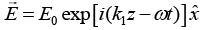over an interface at z = 0 separating two media [wave velocities v1 and v2 (v2 > v1) and wave vectors k1 and k2, respectively] as shown in figure. The magnetic field vector of the reflected wave is (ω is the angular frequency)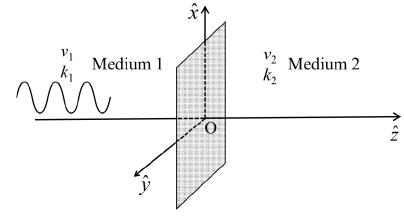a)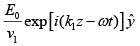b)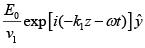c)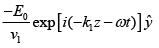d)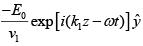Correct answer is option 'C'. Can you explain this answer? Related Test: Physics - 2019 Past Year Paper

## Can you answer this question?

People are searching for an answer to this question.